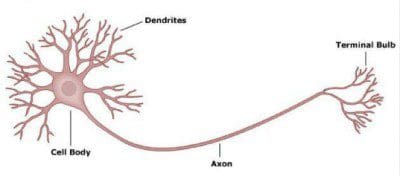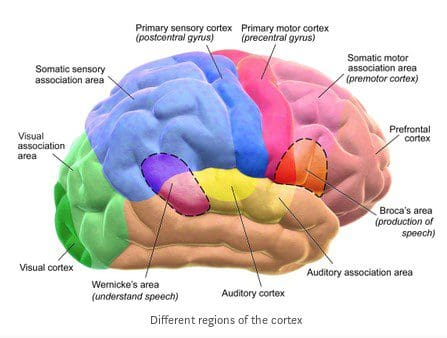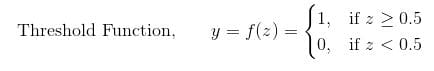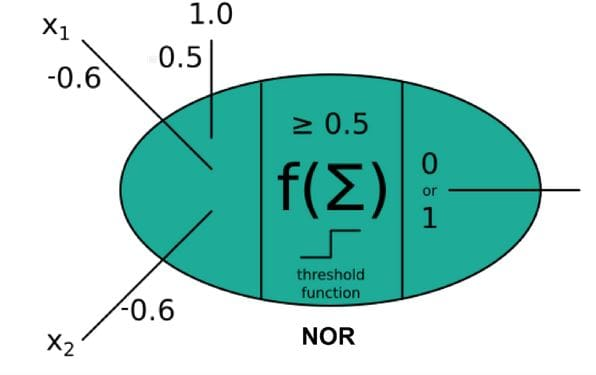Submit a blog to KDnuggets -- Top Blogs Win A Reward

Topics: AI | Data Science | Data Visualization | Deep Learning | Machine Learning | NLP | Python | R | Statistics

KDnuggets Home » News » 2019 » Mar » Tutorials, Overviews » Neural Networks with Numpy for Absolute Beginners: Introduction ( 19:n10 )

# Neural Networks with Numpy for Absolute Beginners: Introduction

In this tutorial, you will get a brief understanding of what Neural Networks are and how they have been developed. In the end, you will gain a brief intuition as to how the network learns.comments

By Suraj Donthi, Computer Vision Consultant & Course Instructor at DataCamp

Artificial Intelligence has become one of the hottest fields in the current day and most of us willing to dive into this field start off with Neural Networks!!

But on confronting the math intensive concepts of Neural Networks we just end up learning a few frameworks like Tensorflow, Pytorch etc., for implementing Deep Learning Models.

Moreover, just learning these frameworks and not understanding the underlying concepts is like playing with a black box.

Whether you want to work in the industry or academia, you will be working, tweaking and playing with the models for which you need to have a clear understanding. Both the industry and the academia expect you to have full clarity of these concepts including the math.

In this series of tutorials, I’ll make it extremely simple to understand Neural Networks by providing step by step explanation. Also, the math you’ll need will be the level of high school.

Let us start with the inception of artificial neural networks and gain some inspiration as to how it evolved.

### A little bit into the history of how Neural Networks evolved

It must be noted that most of the Algorithms for Neural Networks that were developed during the period 1950–2000 and now existing, are highly inspired by the working of our brain, the neurons, their structure and how they learn and transfer data. The most popular works include the Perceptron(1958) and the Neocognitron(1980). These papers were extremely instrumental in unwiring the brain code. They try to mathematically formulate a model of the neural networks in our brain.

And everything changed after the God Father of AI Geoffrey Hinton formulated the back-propagation algorithm in 1986(That’s right! what you are learning is more than 30 years old!).

### A biological NeuronThe figure above shows a biological neuron. It has dendrites that receive information from neurons. The received information is passed on to the cell body or the nucleus of the neuron. The nucleus is where the information is processed and passed on to the next layer of neurons through axons.

Our brain consists of about 100 billion such neurons which communicate through electrochemical signals. Each neuron is connected to 100s and 1000s of other neurons which constantly transmit and receive signals.

But how can our brain process so much information just by sending electrochemical signals? How can the neurons understand which signal is important and which isn't? How do the neurons know what information to pass forward?

The electrochemical signals consist of strong and weak signals. The strong signals are the ones to dominate which information is important. So only the strong signal or a combination of them pass through the nuclues (the CPU of neurons) and are transmitted to the next set of neurons through the axons.

But how are some signals strong and some signals week?

Well, through millions of years of evolution, the neurons have become sensitive to certain kinds of signals. When the neuron encounters a specific pattern, they get triggered(activated) and as a consequence send strong signals to other neurons and hence the information is transmitted.

Most of us also know that different regions of our brain are activated (or receptive) for different actions like seeing, hearing, creative thinking and so on. This is because the neurons belonging to a specific region in the brain are trained to process a certain kind of information better and hence get activated only when certain kinds of information is being sent. The figure below gives us a better understanding of the different receptive regions of the brain.If that is so… can the neurons be made sensitive to a different pattern(i.e., if they have truly become sensitive based on some patterns)?

It has been shown through Neuroplasticity that the different regions of the brain can be rewired to perform totally different tasks. Such as the neurons responsible for touch sensing can be rewired to become sensitive to smell. Check out this great TEDx video below to know more about neuroplasticity.

TEDx video on Neuroplasticity [Source]

But what is the mechanism by which the neurons become sensitive?

Unfortunately, neuroscientists are still trying to figure that out!!

But fortunately enough, god father of AI Geff has saved the day by inventing back propagation which accomplishes the same task for our Artificial Neurons, i.e., sensitizing them to certain patterns.

In the next section, we’ll explore the working of a perceptron and also gain a mathematical intuition.

### Perceptron/Artificial NeuronFrom the figure, you can observe that the perceptron is a reflection of the biological neuron. The inputs combined with the weights(wᵢ) are analogous to dendrites. These values are summed and passed through an activation function (like the thresholding function as shown in fig.). This is analogous to the nucleus. Finally, the activated value is transmitted to the next neuron/perceptron which is analogous to the axons.

The latent weights(wᵢ) multiplied with each input(xᵢ) depicts the significance(strength) of the respective input signal. Hence, larger the value of a weight, more important is the feature.

You can infer from this architecture that the weights are what is learned in a perceptron so as to arrive at the required result. An additional bias(b, here w₀) is also learned.

Hence, when there are multiple inputs (say n), the equation can be generalized as follows:

Finally, the output of summation (assume as z) is fed to the thresholding activation function, where the function outputs

### An ExampleLet us consider our perceptron to perform as logic gates to gain more intuition.

Let’s choose an AND Gate. The Truth Table for the gate is shown below:

The perceptron for the AND Gate can be formed as shown in the figure. It is clear that the perceptron has two inputs (here x₁ = A and x₂ = B).We can see that for inputs x₁, x₂ and x₀ = 1, setting their weights as

respectively and keeping the Threshold function as the activation function we can arrive at the AND Gate.

Now, let’s get our hands dirty and codify this and test it out!

def and_perceptron(x1, x2):

w0 = -0.5
w1 = 0.6
w2 = 0.6

z = w0 + w1 * x1 + w2 * x2

thresh = lambda x: 1 if x>= 0.5 else 0

r = thresh(z)
print(r)

>>>and_perceptron(1, 1)

1

Similarly for NOR Gate the Truth Table is,The perceptron for NOR Gate will be as below:You can set the weights as

so that you obtain a NOR Gate.
You can go ahead and implement this in code as:

def nor_perceptron(x1, x2):

w0 = 0.5
w1 = -0.6
w2 = -0.6

z = w0 + w1 * x1 + w2 * x2

thresh = lambda x: 1 if x>= 0.5 else 0

r = thresh(z)
print(r)

>>>nor_perceptron(1, 1)

0

### What you are actually calculating…

If you analyse what you were trying to do in the above examples, you will realize that you were actually trying to adjust the values of the weights to obtain the required output.

Lets consider the NOR Gate example and break it down to very miniscule steps to gain more understanding.

What you would usually do first is to simply set some values to the weights and observe the result, say

Then the output will be as shown in below table:So, how can you fix the values of weights so that you get the right output?

By intuition, you can easily observe that w₀ must be increased and w₁ and w₀ must be reduced or rather made negative so that you obtain the actual output. But if you breakdown this intuition, you will observe that you are actually finding the difference between the actual output and the predicted output and finally reflecting that on the weights…

This is a very important concept that you will be digging deeper and will be the core to formulate the ideas behind gradient descent and also backward propagation.

### What did you learn?

• Neurons must be made sensitive to a pattern in order to recognize it.
• So, similarly, in our perceptron/artificial neuron, the weights are what is to be learnt.

In the later articles you’ll fully understand how the weights are trained to recognize patterns and also the different techniques that exist.

As you’ll see later, the neural networks are very similar to the structure of biological neural networks.

While it is true that we learnt only a few small concepts (although very crucial) in this first part of the article, they will serve as the strong foundation for implementing Neural Networks. Moreover, I’m keeping this article short and sweet so that too much is information is not dumped at once and will help absorb more!

In the next tutorial, you will learn about Linear Regression (which can otherwise be called a perceptron with linear activation function) in detail and also implement them. The Gradient Descent algorithm which helps learn the weights are described and implemented in detail. Lastly, you’ll be able to predict the outcome of an event with the help of Linear Regression. So, head on to the next article to implement it!

You can checkout the next part of the article here:

Neural Networks with Numpy for Absolute Beginners — Part 2: Linear Regression

Bio: Suraj Donthi is a Computer Vision Consultant, Author, Machine Learning and Deep Learning Trainer.

Original. Reposted with permission.

Related:

## Top Stories Past 30 Days

Most PopularGet KDnuggets, a leading newsletter on AI, Data Science, and Machine Learning

By subscribing you accept KDnuggets Privacy Policy